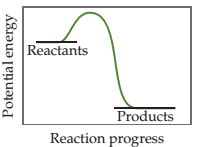Create an Account

Home / Questions / a) Based on the following energy profile, predict whether kf ˃ kr or kf < kr. (b) Using Eq...

a) Based on the following energy profile, predict whether kf ˃ kr or kf < kr. (b) Using Equation 15.5, predict whether the equilibrium constant for the process is greater than 1 or less than

a) Based on the following energy profile, predict whether kf ˃ kr or kf < kr(b) Using Equation 15.5, predict whether the equilibrium constant for the process is greater than 1 or less than 1.Aug 21 2021 View more View LessSubscribe To Get Solution